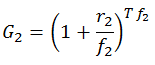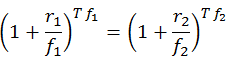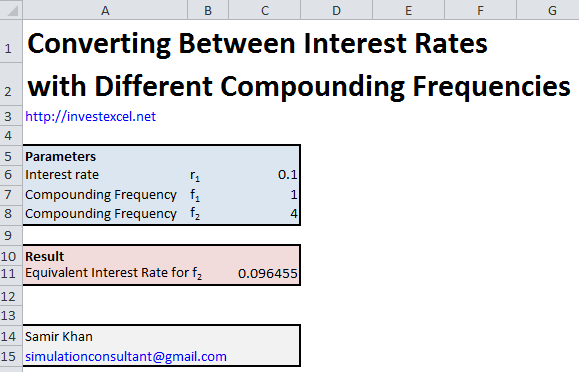# Convert Between Interest Rates with Different Compounding Frequencies

Learn how to convert between interest rates with different compounding frequencies.

Many financial products accrue interest at different rates.

For example, savings accounts offered by different banks may not have the same compounding frequency. Additionally, mortgages offered in some countries accrue interest once per year, while other countries have higher compounding frequencies.

Assume that you have an interest rate of r1, invested for T years and a compounding frequency of f1 times per year. The total amount accrued after T years isLets say that you want to calculate the equivalent interest rate for a compounding frequency of f2. The amount accrued under the new compounding frequency isHowever, G1 = G2, and henceRearranging this equation gives a formula for r2Note that r2 has no dependence on the investment time T.